# Solution assignment 03 Quadratic functions and graphs

### Assignment 3

Given the function: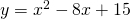1. What is the intersection point of the graph with the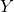-axis?
2. Does the graph have intersection points with the-axis, and if so, how many? Calculate the coordinates.
3. What is the symmetry axis?

### Solution

1. Because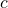in the formula equals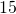, the intersection point with the-axis equals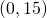.

2. In order to find out whether the graph of the function has intersection points with the-axis, we have to calculate the discriminant. However, we can also notice that the equation can be factorized: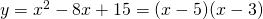Then we kill two birds with one stone. There are two intersection points with the-axis and their coordinates are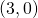and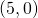.

3. The symmetry axis lies just in the middle of these two points and thus the symmetry axis is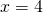.

0